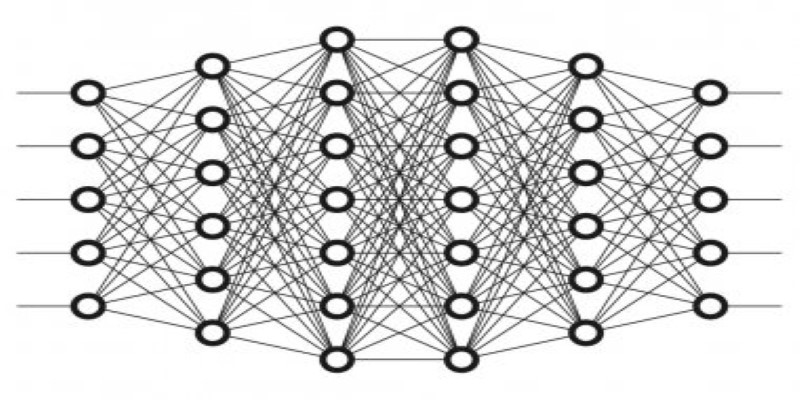# Neural Network using MATLAB in 2020

Wishlist Share
Share Course
Share On Social Media### Neural Network using MATLAB in 2020

This course provides a comprehensive introduction to the neural network for the data fitting problems using MATLAB. Attendees will learn to construct, train, and simulate different kinds of neural networks. A set of practical problems are solved in this course. At the end of this course, you will be able to solve the Neural Network problems using the MATLAB – Neural Network Toolbox. The MATLAB scripts and functions included in the class are also available for download. Happy learning.

NB: This course is designed most straightforwardly to utilize your time wisely.

## Who this Neural Network using MATLAB course is for:

1. Those who would like to build a strong career in science/engineering through MATLAB programming skills.

### What Will You Learn?

• Introductory Concepts of Neural Network
• Construction, Training, and Simulation of Neural Network in MATLAB ToolBox
• Gain Hands-On Experience With MATLAB Neural Network Programming

00:00
00:00
00:00
00:00
00:00
00:00
00:00
00:00
00:00
00:00
00:00
00:00
00:00
00:00
00:00
00:00
00:00
00:00

### Student Ratings & ReviewsNo Review Yet